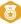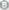﻿ 有个颜色值它的r,g,b各占8位如:r=0xf0, g=0xf8, b=0xff 我现在想把它的r,g,b分别扩展到16位 | Code Bye

# 有个颜色值它的r,g,b各占8位如:r=0xf0, g=0xf8, b=0xff 我现在想把它的r,g,b分别扩展到16位

5年前 (2015-04-25) 316次浏览
 有个颜色值它的 r,g,b各占8位如 :r=0xf0, g=0xf8, b=0xff  我现在想把它的r,g,b分别扩展到16位(0xffff代表满值,0x0000代表无值),请问怎么计算!谢谢,好像不是太难,但是我想不出个好办法. 要代码100分谢谢5分 r、g、b 是各占2位 前面的0x代表是16进制 笨方法： ``` public String getRGBToString(int r,int g,int b){ int rgb=0; rgb=r*(int)Math.pow(16, 4)+g*(int)Math.pow(16, 2)+b; return Integer.toHexString(rgb).toUpperCase();//16进制字符串形式 } public int getRGBToInt(int r,int g,int b){ int rgb=0; rgb=r*(int)Math.pow(16, 4)+g*(int)Math.pow(16, 2)+b; return rgb;//默认输出是10进制 }```那就是说要改变最大值了被。。。。。比如说int r = 0xfo  那么int newr =r*16*16*16*16   这就是改变范围了啊。。。。引用 3 楼 u013280307 的回复: 那就是说要改变最大值了被。。。。。比如说int r = 0xfo  那么int newr =r*16*16*16*16   这就是改变范围了啊。。。。 我的意思是它原来是８位，现在要扩展到１６位。你说的我看不明白。。。引用 2 楼 inquisitive_plus 的回复: r、g、b 是各占2位 前面的0x代表是16进制 笨方法： ``` public String getRGBToString(int r,int g,int b){ int rgb=0; rgb=r*(int)Math.pow(16, 4)+g*(int)Math.pow(16, 2)+b; return Integer.toHexString(rgb).toUpperCase();//16进制字符串形式 } public int getRGBToInt(int r,int g,int b){ int rgb=0; rgb=r*(int)Math.pow(16, 4)+g*(int)Math.pow(16, 2)+b; return rgb;//默认输出是10进制 }``` 引用 2 楼 inquisitive_plus 的回复: r、g、b 是各占2位 前面的0x代表是16进制 笨方法： ``` public String getRGBToString(int r,int g,int b){ int rgb=0; rgb=r*(int)Math.pow(16, 4)+g*(int)Math.pow(16, 2)+b; return Integer.toHexString(rgb).toUpperCase();//16进制字符串形式 } public int getRGBToInt(int r,int g,int b){ int rgb=0; rgb=r*(int)Math.pow(16, 4)+g*(int)Math.pow(16, 2)+b; return rgb;//默认输出是10进制 }``` 不是，我没说清，我的意思是，不是要rgb的值，只把单一的一个分量值。比如r值，它原来是８位它的取值范围是0x00-0xff，（值越大代表r颜色越饱满）。现在我想把这个８位的r值扩展成１６位来表示， 这样它的取值范围就变成了0x0000-0xffff，那么还要接近原来的颜色效果。怎么扩展比较好？谢谢rgb565就是个等比运算啊 a/b = (2^16)/(2^8) a = (2^16)/(2^8) * b直接就各乘两个16就可以了啊。。。。等比扩大。。。小学生都懂的引用 7 楼 assky124 的回复: 就是个等比运算啊 a/b = (2^16)/(2^8)a = (2^16)/(2^8) * b 能不能按需要给用java写个函数出来，90分上都是你的。劳驾谢谢！95分 试试 ```    public static long toLargeRgb(int color){         long a = Color.alpha(color);         long r = Color.red(color);         long g = Color.green(color);         long b = Color.blue(color);         return a<<(48+8)|r<<(32+8) | g<<(16+8) | b<<8;     }```0xffff0000 转换为0xff00ff0000000000

CodeBye 版权所有丨如未注明 , 均为原创丨本网站采用BY-NC-SA协议进行授权 , 转载请注明有个颜色值它的r,g,b各占8位如:r=0xf0, g=0xf8, b=0xff 我现在想把它的r,g,b分别扩展到16位

[1034331897@qq.com]